# Object Property Initializer Shorthand in JavaScript

## Object Property Initializer Shorthand in JavaScript with Examples

In this article, I am going to discuss Object Property Initializer Shorthand / Short Properties / Property Value Shorthand’s /Duplicate Property Names /Object Initialization from Variables in JavaScript with Examples. Please read our previous article where we discussed How to Find the Length of a JavaScript Object.

Before ES6, an object literal is a list of name-value pairs comma-separated inside of curly braces. In ES6 Whenever we assign a property value that matches a property name, we can omit the property value, it’s implicit (automatic) in ES6.

As ES6 allows to remove the duplication when a property value of an object is the same as the variable name/property name by including the name without the colon and value.

When using the same name for properties, the second property will overwrite the first one. This is called duplicate property name removal features of object literals. Objects’ properties are often created from variables with the same name. it is called Object Initialization from variables.

###### Example: JavaScript Object Literal-Property Shorthand
```<html>
<title>JavaScript Object Literal-Property shorthand example</title>
<body>
<script>
//before ES6 old syntax
const x = 10, y = 20;
const oldObj = {
x: x,
y: y
}
console.log("Before ES6- Property Shorthand: ", oldObj)
console.log("Before ES6- Property Shorthand: " + "value of x: " + oldObj.x + " value of y: " + oldObj.y)

//with ES6 new syntax
const a = 10, b = 20;
const newObj = {
a, b
}
console.log("With ES6- Property Shorthand: ", newObj)
console.log("With ES6- Property Shorthand: " + "value of a: " + newObj.a + " value of b: " + newObj.b)

//same name for properties, the second property will overwrite the first.
let c = { z: 1, z: 2 }
console.log("Same name for properties, the second property will overwrite the first: ", c); // {z: 2}
</script>
</body>
</html>
```

Output: Now run the above code and open the browser developer tool and select the Console tab and you will see the following output.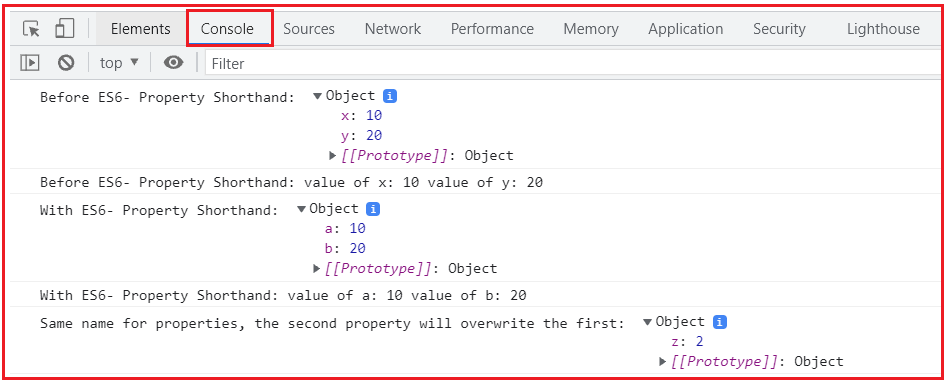##### Computed Property Names/Dynamic Property Keys in JavaScript

Prior to ES6 in ES5 and earlier, we could not use a variable as a property name inside an object literal. The only way we had was to create the object literal then assign the variable property name with value and pass the resulting object to the animate method.

ES6 defines ‘ComputedPropertyName’ as part of the object literals, which helps use a variable for an object key. Object keys can be dynamically assigned in ES6 by placing an expression in square brackets [ ].

Computed property names allow us to write an expression enclosed in square brackets [ ] instead of the regular property name. Whatever the expression is it will be computed and will be used as the property name.

Property names that are not valid identifiers cannot be accessed as an object name dot (.) property but can be accessed and set the property name with the array-like notation (“[]”).

The limitation of computed property names is that we won’t be able to use the shorthand expression with it. Other types are automatically converted to strings.

###### Syntax: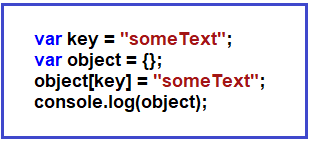A dynamic key can be used for methods as well as properties.

###### Example1: JavaScript Object Literal-Computed Property Names
```<html>
<title>JavaScript Object Literal-Computed Property Names example</title>
<body>
<script>
//before ES6 old syntax
const propertyName = 'Property1';
const computed_property_Name = () => 'Property-3*' + 3;

const oldObj = {};
oldObj[propertyName] = 'string';
oldObj[computed_property_Name()] = 3 * 3;
console.log("Before ES6- Computed Property Names: ", oldObj)

//With ES6 new syntax
const propName = 'Prop1';
const computed_prop_Name = () => 'Property-3*' + 3;

const newObj = {
[propName]: 'str',
[computed_prop_Name()]: 3 * 3
};
console.log("With ES6- Computed Property Names: ", newObj)

</script>
</body>
</html>
```

Output: Now run the above code and open the browser developer tool and select the Console tab and you will see the following output.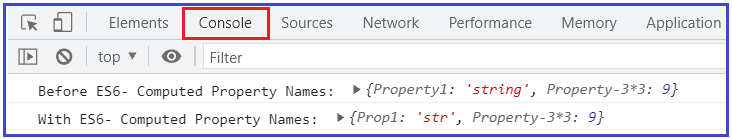Any expression can be used to create a key. For example, below sample code no-4 elaborate.

###### Example2: JavaScript Object Literal-Computed Property Names
```<html>
<title>JavaScript Object Literal-Computed Property Names Example</title>
<body>
<script>
//sample1 code
var key = "Dynamic Property Names";
var object = {};
object[key] = "JavaScript";
console.log("With ES6- Computed Property Names-sample code1: ", object)

//sample2 code
var key1 = "Dynamic Property Names";
var object1 = {
[key1]: "JavaScript"
};
console.log("With ES6- Computed Property Names-sample code2: ", object1)

//sample3 code
let name = 'Weekday name';
let Week = {
[name]: 'Monday',
'day hours': 24
};
console.log("With ES6- Computed Property Names-sample code3: ", Week)
console.log('Week name is: ', Week[name]); // Monday
console.log('Day hours is ', Week['day hours']); // 24

//sample4 code
let i = 0
let dynamicObjKey = {
['welcome' + ++i]: i,
['welcome' + ++i]: i,
['welcome' + ++i]: i
}
console.log("With ES6- Computed Property Names-sample code4: ", dynamicObjKey)
console.log('dynamicObjKey welcome1 value: ', dynamicObjKey.welcome1) // 1
console.log('dynamicObjKey welcome1 value: ', dynamicObjKey.welcome2) // 2
console.log('dynamicObjKey welcome1 value: ', dynamicObjKey.welcome3) // 3

//sample5 code
const items = ["Notebook", "Pen", "Laptop"];
const obj = {
[items]: "Item Lists"
}
console.log("With ES6- Computed Property Names-sample code5: ", obj)//Notebook,Pen,Laptop: "Item Lists"
console.log('obj items key value is: ',obj[items]) // Item Lists
</script>
</body>
</html>
```

Output: Now run the above code and open the browser developer tool and select the Console tab and you will see the following output.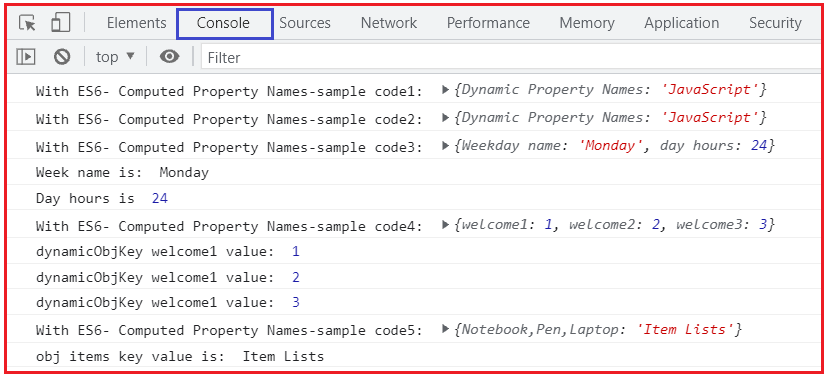JavaScript ES6 provides a way to use variables as object keys — we have to wrap the key in square brackets ([]) also called Computed property names. We can also access the object properties by using a string value that is stored in a variable.

```const tutorial = 'course';
let lecture = {
[tutorial]: 'JavaScript',
version: 'ES6'
}
console.log(lecture); //{course: "JavaScript", version: "ES6"}
console.log(lecture['course']); //JavaScript-bracket notion
console.log(lecture.course); //JavaScript- dot notation
console.log(lecture[tutorial]); //JavaScript
console.log(lecture['tutorial']); //undefined
console.log(lecture.tutorial); //undefined
```
##### Use Variable as Object Key/ Computed Property Names in JavaScript

Similarly, if we have a variable const tutorial = ‘course’ and try to create an object with the variable as the object key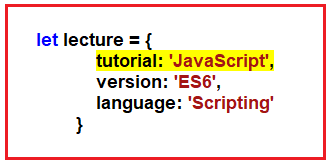The lecture object’s key would be ‘tutorial’, not ‘course‘. What we discussed above is given in the below example.

###### Example: JavaScript Object, using a variable as the object key
```<html>
<title>JavaScript Object, using a variable as object key example</title>
<body>
<script>
const tutorial = 'course';

let lecture = {
tutorial: 'JavaScript',
version: 'ES6',
language: 'Scripting'
}

console.log('JavaScript Simple Object, using variable as object key:\n ', lecture);
console.log('Type of variable lecture: ', typeof lecture)

</script>
</body>
</html>
```

Output: Now run the above code and open the browser developer tool and select the Console tab and you will see the following output.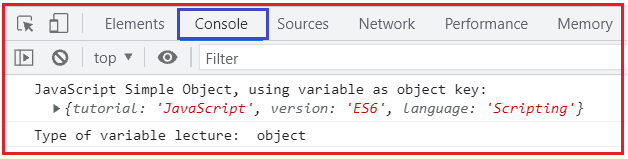Best Tip: JavaScript ES6 provides a way to use variables as object keys — we have to wrap the key in square brackets ([]) also called Computed property names. Using the above example, we could write const tutorial = ‘course’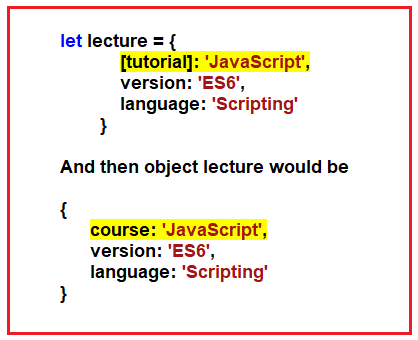Pretty cool, isn’t it? What we discussed above is given in the below example.

###### Example: JavaScript Object, wrap the key in square brackets to use the variable as the object key
```<html>
<title>JavaScript Object, wrap the key in square brackets to use variable as object key (Computed property names) example</title>
<body>
<script>
const tutorial = 'course';

let lecture = {
[tutorial]: 'JavaScript',
version: 'ES6',
language: 'Scripting'
}

console.log('JavaScript Simple Object, wrap the key in square brackets to use variable as object key:\n ', lecture);
console.log('Type of variable lecture: ', typeof lecture)

</script>
</body>
</html>
```

Output: Now run the above code and open the browser developer tool and select the Console tab and you will see the following output.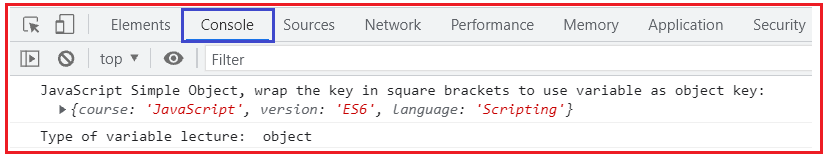We can also access the object properties by using a string value that is stored in a variable. What we discussed above is given in the below example.

###### Example: JavaScript Object, access the object properties by using a string value that is stored in a variable
```<html>
<title>JavaScript Object, access the object properties by using a string value that is stored in a variable example</title>
<body>
<script>
const tutorial = 'course';

//sample code1
//initialize an object with key-value pairs, create an object
console.log('===sample code1 with initialize an object with key-value pairs syntax===');
let lecture = {
[tutorial]: 'JavaScript',
version: 'ES6',
language: 'Scripting'
}
console.log('JavaScript Simple Object, wrap the key in square brackets to use variable as object key:\n ', lecture);
console.log("Object Literal - lecture object length: ", Object.getOwnPropertyNames(lecture).length);
console.log('Type of variable lecture: ', typeof lecture)
console.log('JavaScript Object, access the object properties by using a string value(course) that is stored in a variable, with bracket notation:', lecture['course']); //JavaScript
console.log('JavaScript Object, access the object properties by using a string value(course) that is stored in a variable, with dot notation:', lecture.course); //JavaScript
console.log('JavaScript Object, access the variable(tutorial) which is set as object key, with bracket notation:', lecture[tutorial]); //JavaScript
console.log('JavaScript Object, access the variable(tutorial) which is set as object key, quoted property name with bracket notation:', lecture['tutorial']);//undefined
console.log('JavaScript Object, access the variable(tutorial) which is set as object key, with dot notation:', lecture.tutorial); //undefined
//console.log('JavaScript Object, access the  property name(version), with bracket notation:', lecture[version]); // Uncaught ReferenceError: version is not defined
console.log('JavaScript Object, access the  property name(version), quoted property name with bracket notation:', lecture['version']); //ES6

//sample code2
//"object literal" syntax, create an object
console.log('===sample code2 with object literal syntax===');
let lecture1 = {};
lecture1[tutorial] = 'JavaScript';
lecture1.version = 'ES6';
lecture1.language = 'Scripting';

console.log('JavaScript Simple Object, wrap the key in square brackets to use variable as object key:\n ', lecture1);
console.log("Object Literal – lecture1 object length: ", Object.getOwnPropertyNames(lecture1).length);
console.log('Type of variable lecture1: ', typeof lecture1)
console.log('JavaScript Object, access the object properties by using a string value(course) that is stored in a variable, with bracket notation:', lecture1['course']); //JavaScript
console.log('JavaScript Object, access the object properties by using a string value(course) that is stored in a variable, with dot notation:', lecture1.course); //JavaScript
console.log('JavaScript Object, access the variable(tutorial) which is set as object key, with bracket notation:', lecture1[tutorial]); //JavaScript
console.log('JavaScript Object, access the variable(tutorial) which is set as object key, quoted property name with bracket notation:', lecture1['tutorial']);//undefined
console.log('JavaScript Object, access the variable(tutorial) which is set as object key, with dot notation:', lecture1.tutorial); //undefined
//console.log('JavaScript Object, access the  property name(version), with bracket notation:', lecture1[version]); // Uncaught ReferenceError: version is not defined
console.log('JavaScript Object, access the  property name(version), quoted property name with bracket notation:', lecture1['version']); //ES6

</script>
</body>
</html>
```

Output: Now run the above code and open the browser developer tool and select the Console tab and you will see the following output.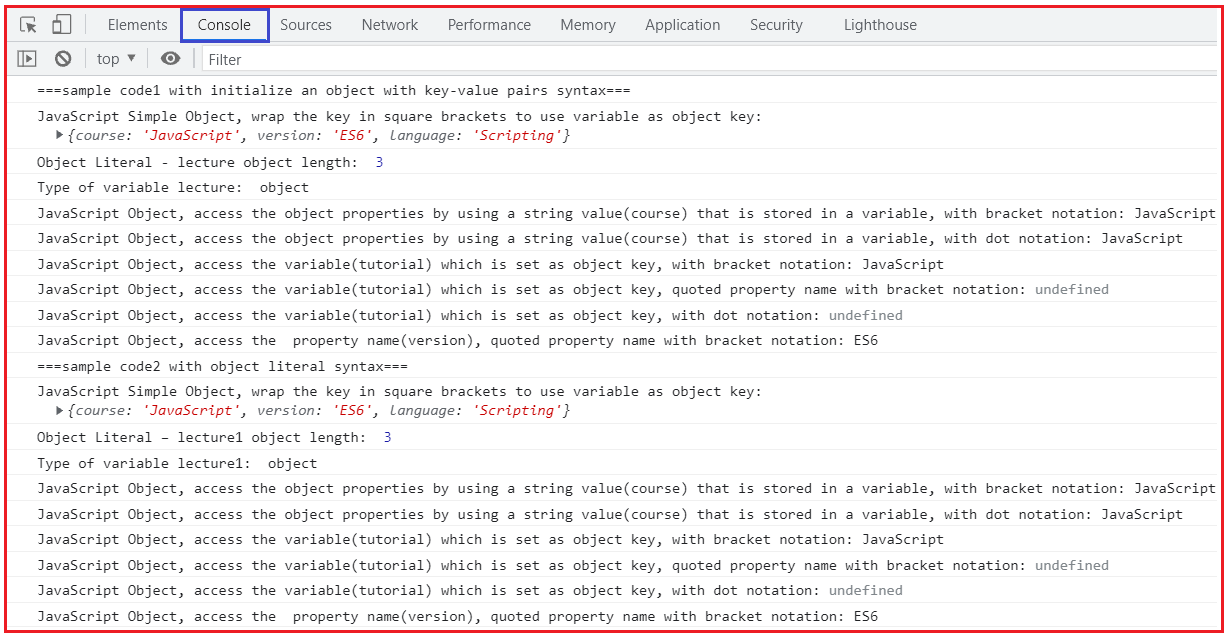In the above example, sample code1 and sample code2 we are creating the object one with literal syntax and the other with key-value pair syntax which we learned already.

In the above when we try to access the object properties i.e.: [tutorial]: ‘JavaScript’, by using a string value(course) that is stored in a variable i.e.: const tutorial = ‘course’; with both bracket notation i.e.: lecture[‘course’] as well as dot notation i.e.: lecture.course it returns the value assigned to it which is JavaScript.

Next, when we try to access the variable(tutorial) i.e.: const tutorial = ‘course’ which is set as object key i.e.: [tutorial]: ‘JavaScript’, with bracket notation i.e.: lecture[tutorial] its returns JavaScript this is because its variable use as object property name.

Whereas when we try to access the normal property name i.e.: version: ‘ES6’, with bracket notation i.e.: lecture[version] it throws an Uncaught ReferenceError: version is not defined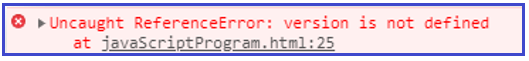Note that when we try to access the variable(tutorial) i.e.: const tutorial = ‘course’ which is set as object key i.e.: [tutorial]: ‘JavaScript’, with bracket notation having property name quoted i.e.: lecture[‘tutorial’] it’s returns undefined. This is because it is a variable that is set as an object key and we are trying to access it like normal property with quoted property name using bracket notation hence it gives undefined.

Whereas when we try to access the normal property name i.e.: version: ‘ES6’, with bracket notation having property name quoted i.e.: lecture[‘version’] it returns ES6.

Similarly, when we try to access the variable(tutorial) which is set as the object key, with dot notation i.e.: lecture.tutorial its returns undefined. Because of a variable that is set as an object key and we are trying to access it with dot notation in turn it gives undefined.

We already learned in earlier chapter Object creation using object literal that JavaScript ES6 provides a way to use variables as object keys — we have to wrap the key in square brackets ([]) also called Computed property names.

The square bracket notation is also very useful when property names are to be dynamically determined (when the property name is not determined until runtime) this is possible by using a variable as an object key and wrapping that key in a square bracket [].

###### Example: Objects creation in JavaScript, by Using new Object() method, wrap the key in square brackets to use a variable as object key called Computed property names
```<html>
<title>Objects creation in JavaScript, by Using new Object() method, wrap the key in square brackets to use a variable as object key called Computed property names example</title>
<body>
<script>
// four variables are created and assigned in a single go, separated by commas
var myObj = new Object(),
str = 'This is sample string',
randNum = Math.random(),
obj = new Object();

//In order to use variable as object key, wrap the object key in square brackets also called Computed property name/dynamically determined
myObj.type = 'Dot notation syntax';
myObj['date created'] = 'String with space';
myObj[str] = 'String value';
myObj[randNum] = 'Random Number';
myObj[obj] = 'Object';
myObj[''] = 'An empty string';

console.log('typeof myObj.type:', typeof myObj.type); //string
console.log("typeof myObj['date created']:", typeof myObj['date created']); //string
console.log('typeof myObj[str]:', typeof myObj[str]); //string
console.log('typeof myObj[randNum]:', typeof myObj[randNum]); //string
console.log('typeof myObj[obj]:', typeof myObj[obj]); //string
console.log("typeof myObj['']:", typeof myObj['']); //string
console.log('The Computed property names/key of myObj object is:\n ', myObj);
</script>
</body>
</html>
```

Output: Now run the above code and open the browser developer tool and select the Console tab and you will see the following output.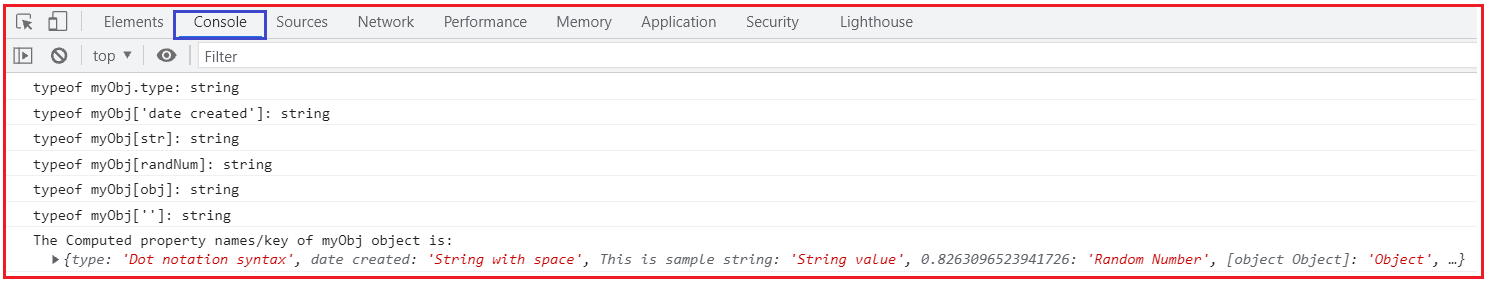In the above-given example, we are using a variable as an object key. So, in order to use a variable as an object key, we have to wrap the object key in square brackets [] which is also called Computed property name/dynamically determined.

Note that all the object keys wrapped in the square bracket notation are cast/converted to string unless they are symbols. That we can see in the above sample where typeof every object key wrapped in a square bracket is a string.

Also, above we are using property names/key data created for myObj object that contains a space i.e.: myObj[‘date created’], we need to use quotes around the property name. That can only be accessed using square bracket notation [].

In this article, I am going to discuss JavaScript Object Property flags and descriptors /Object properties configuration with Examples. Here, in this article, I try to explain Object Property Initializer Shorthand / Short Properties / Property Value Shorthand’s /Duplicate Property Names /Object Initialization from Variables with Examples and I hope you enjoy this Object Property Initializer Shorthand / Short Properties / Property Value Shorthand’s /Duplicate Property Names /Object Initialization from Variables article.